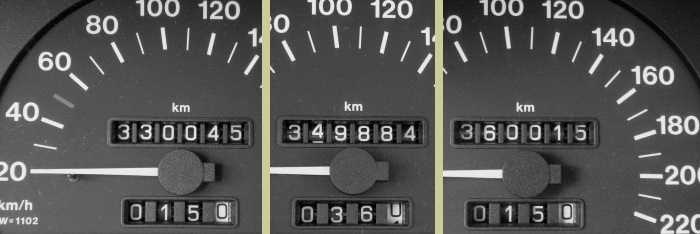SUPER SMITH–NIVEN NUMBERS 1) Let S denote the digital sum: >> 6+6+6 = 18 2) N factors as S times a prime: >> 666 = 18 * 37 3) Let N factor into prime numbers: >> 666 = 2*3*3 * 37 4) Digits of prime factors sum to S: >> 2+3+3 + 3+7 = 18Super Digital Features The number 666, a.k.a. the beast number, obeys three peculiar digital properties: One of its factors is its own digital sum, i.e., 6 + 6 + 6 = 18, and we have the factorization 666 = 18 × 37. The other factor of 37, which is obtained upon dividing 666 by its digital sum, is a prime number, i.e., a number which cannot be factored any further. The complete factorization 666 = 2 × 3 × 3 × 37 shows that the digits of the prime factors also sum to 18, i.e., 6 + 6 + 6 = 2 + 3 + 3 + 3 + 7. A number with Property (1) is called a Niven number, and a number satisfying Property (3) a Smith number. We call a number super Smith–Niven, abbreviated SSN, if it enjoys the above all three digital features shared by the number 666.  The Holy Bible, Revelation XIII v. 18 [KingJames]  Journal of Combinatorics and Number Theory 5 (2013) pp. 215–225 [MathSciNet]Fig. 1: An SSN serial number appearing on a \$100 bank note.Super Digital Sum With this definition, we find that the smallest SSN number is 27, and that there are only fourteen more SSN numbers below ten thousand, including the beast number, i.e., 645, 666, 915, 1962, 2265, 2286, 2934, 3258, 3345, 3615, 5526, 6315, 9015, 9414 Notice that all these numbers have digital sums either 15 or 18. This is a fact, that SSN numbers cannot have an arbitrary digital sum. Except for 27, which has digital sum 9, the digital sum of an SSN number is constrained to a set of values partially listed below: 15, 18, 33, 36, 45, 48, 51, 54, 63, 69, 84, 87, 90, 98, 99, …Fig. 2: Three successive SSN numbers showing on the odometer with their respective digital sum.Observation Tables We conjecture that SSN numbers are infinitely many, although they are relatively rare. For instance, in the interval up to a billion, we identify only about 150,000 SSN numbers, making an average of one SSN in every 6666 natural numbers! We can also ask many stimulating questions that call for further investigation on SSN numbers, e.g., where their distribution is concerned. For research purposes, we have made available a collection of computational output in the following files. The preceding list of super digital sums, extended up to 104 (txt file). The list of all SSN numbers up to 107 (txt file) and up to 109 (zip file 0.5 Mb). The list of SSN primes, i.e., the prime numbers appearing in Property (2), compiled up to 106 (txt file) and up to 109 (zip file 10 Mb).